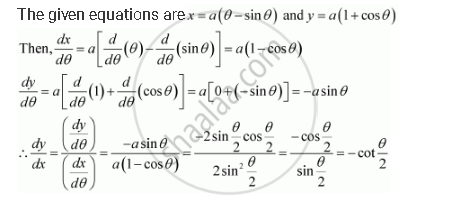Share

# If X And Y Are Connected Parametrically by the Equation, Without Eliminating the Parameter, Find Dy/DxX = a (θ – Sin θ), Y = a (1 + Cos θ) - CBSE (Commerce) Class 12 - Mathematics

ConceptDerivatives of Functions in Parametric Forms

#### Question

If x and y are connected parametrically by the equation, without eliminating the parameter, find dy/dx

x = a (θ – sin θ), y = a (1 + cos θ)

#### SolutionIs there an error in this question or solution?

#### APPEARS IN

NCERT Solution for Mathematics Textbook for Class 12 (2018 to Current)
Chapter 5: Continuity and Differentiability
Q: 6 | Page no. 181
Solution If X And Y Are Connected Parametrically by the Equation, Without Eliminating the Parameter, Find Dy/DxX = a (θ – Sin θ), Y = a (1 + Cos θ) Concept: Derivatives of Functions in Parametric Forms.
S